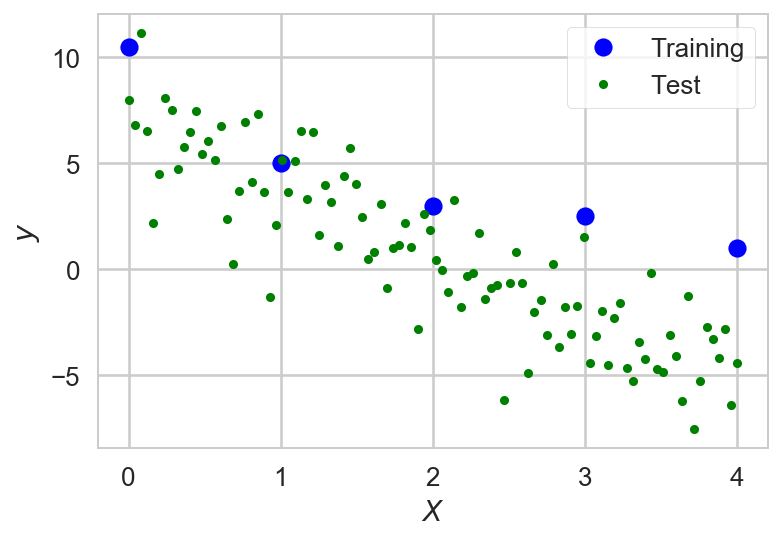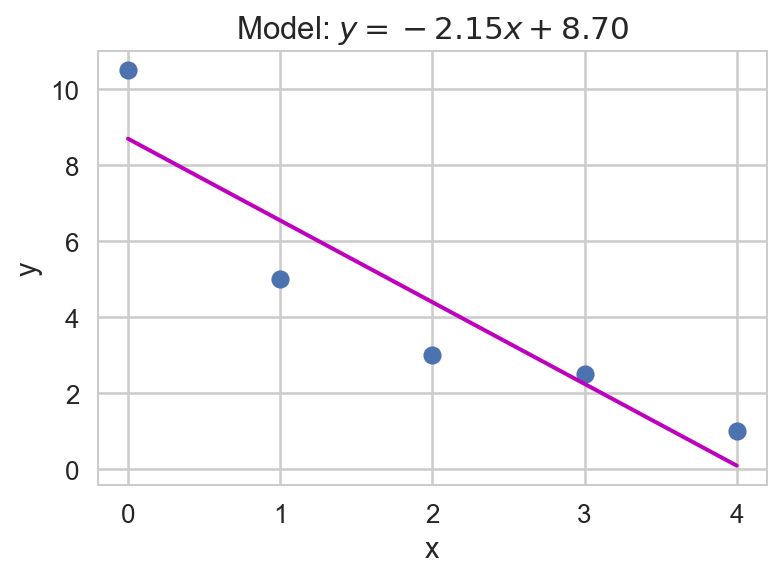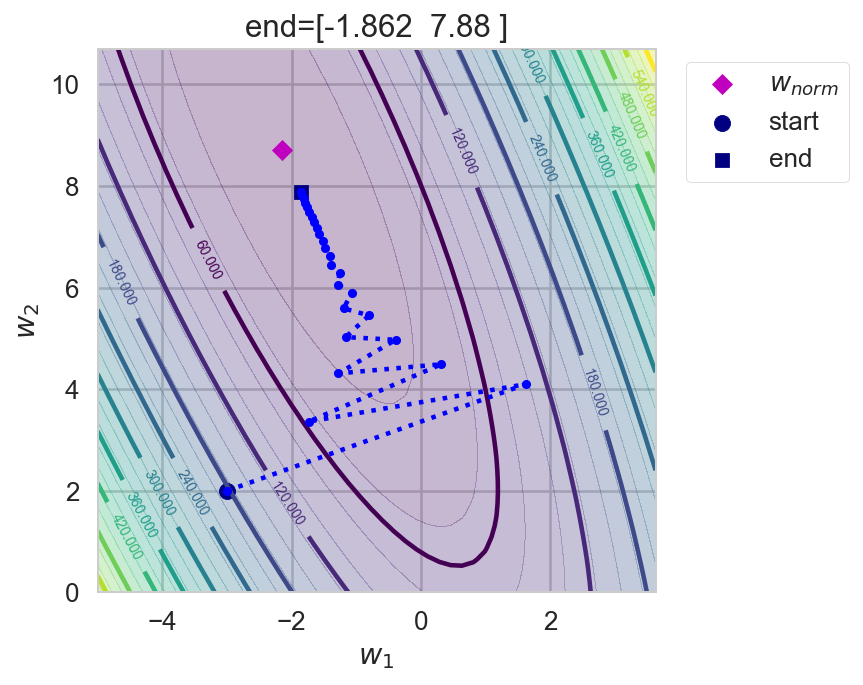# 2. Linear Regression¶

### Luis Martí¶

#### Instituto de Computação¶

If you are using nbviewer you can change to slides mode by clicking on the icon:In :
import random, math
import numpy as np
import matplotlib.pyplot as plt
import matplotlib.cm as cm

In :
#plt.rc('text', usetex=True)
plt.rc('font', family='serif')
#plt.rc('text.latex', preamble='\\usepackage{libertine}\n\\usepackage[utf8]{inputenc}')

# numpy - pretty matrix
np.set_printoptions(precision=3, threshold=1000, edgeitems=5, linewidth=80, suppress=True)

import seaborn
seaborn.set(style='whitegrid'); seaborn.set_context('talk')

%matplotlib inline
%config InlineBackend.figure_format = 'retina'

In :
# Fixed seed to make the results replicable - remove in real life!
random.seed(42)


# Regression$\renewcommand{\vec}{\boldsymbol{#1}}$¶

• We are already familiar with regression problems.
• We have a dataset $\Psi=\left\{\left<\vec{x}^{(1)},\vec{y}^{(1)}\right>,\ldots,\left<\vec{x}^{(i)},\vec{y}^{(i)}\right>,\ldots\left<\vec{x}^{(m)},\vec{y}^{(m)}\right>\right\}$ of noisy samples drawn from and unknown function $\vec{F}:\mathcal{D}\rightarrow\mathcal{I}$.
• In the regression case $\mathcal{D}\subseteq\mathbb{R}^n$ and $\mathcal{I}\subseteq\mathbb{R}^k$.

We can assume that $k=1$ and noise is Gaussian and that $\Psi$ is finite.

### Why we use the Gaussian noise assumption¶

We will assume that the target variable is described by

$$y = \vec{F}(\vec{x},\vec{w}) + \varepsilon$$

where:

• $\vec{F}(\vec{x},\vec{w})$ is a (possibly undefined and/or unknown) function of $\vec{x}$ and $\vec{w}$ and
• $\varepsilon\sim\mathcal{N}(0,\sigma^2_\varepsilon)$ is a Gaussian-distributed noise component.

#### Gaussian Noise?¶

The derivations provided below all assume Gaussian noise on the target data. Is this a good assumption? In many cases yes. The argument hinges on the use of the Central_Limit_Theorem that basically says the the sum of many independent random variables behaves behaves like a Gaussian distributed random variable.

The noise term in this model, $\epsilon$, can be thought of as the sum of features not included in the model function, $\vec{F}(\vec{x},\vec{w})$. Assuming these features are themselves independent random variables then the Central Limit Theorem suggests a Gaussian model is appropriate, assuming there are many independent unaccounted for features.

It is possible that there is only a small number of unaccounted for features or that there is genuine non-Gaussian noise in our observation measurements, e.g. sensor shot noise that often has a Poisson distribution. In such cases, the assumption is no longer valid.

# An Example¶

Training dataset

In :
N = 5                                              # Size of the data set
X = np.array([[0.0], [1.0], [2.0], [3.0], [4.0]])  # Inputs, shape: N x 1
y = np.array([10.5, 5.0, 3.0, 2.5, 1.0])           # Outputs, shape: N

In :
X.T

Out:
array([[ 0.,  1.,  2.,  3.,  4.]])
In :
y

Out:
array([ 10.5,   5. ,   3. ,   2.5,   1. ])

Test dataset

In :
N_test = 100
X_test = np.linspace(0.0, 4.0, N_test).reshape((N_test, 1))
y_test = np.linspace(7.0, -5.0, N_test)+ 2*np.random.randn(N_test)


### Ploting the training and test datasets¶

In :
plt.plot(X[:, 0], y, 'bo', label='Training')
plt.plot(X_test[:, 0], y_test, "g.", label='Test')
plt.xlabel('$X$'); plt.ylabel('$y$'); plt.legend(frameon=True);# Linear Regression Model¶

• We can express our constraints down with a linear model:
$$y^{(i)} + \varepsilon^{(i)} = \vec{x}^{(i)}\cdot \vec{w} + b, \forall i \in \{1,\ldots,m\}\,,$$

where $\vec{w}$ and $b$ constitute a weight vector and $\varepsilon^{(n)} \sim \mathcal{N}(0, \sigma^2_\varepsilon)$.

• Weights are parameters of the linear model that can be tuned to fit the training data better.

A shorter version of this expression is the following equation:

$$\vec{y} + \vec{\epsilon} = \vec{X} \cdot \vec{w},\ \vec{X} \in \mathbb{R}^{m \times n},\ \vec{y} \in \mathbb{R}^m,\ \vec{\epsilon} \in \mathbb{R}^m,\ \vec{w} \in \mathbb{R}^n,$$

where the $i$-th row of $\vec{X}$ represents $\vec{x}^{(i)}$ and the $j$-th entry of the vector $\vec{y}$ represents $\vec{y}^{(j)}$.

• It might not be possible to find a weight vector that satisfies the constraints perfectly.
• Instead, we can minimize the sum of squared errors (SSE):
$$\hat{\vec{w}} = \text{arg}\,\text{min}_\vec{w} \dfrac{1}{2}\left\|\vec{X}\vec{w} - \vec{y}\right\|^2_2,$$

where $\left\|\vec{A}\right\|_2$ is called Frobenius norm and is the generalization of the Euclidean norm for matrices.

The frequentist (maximum likelihood) approach

• assumes that we first obtain observation training data, $\vec{y}$, and that the best value of $\vec{w}$, is that which maximizes the likelihood function, $p(\vec{y}|\vec{x},\vec{w})$.

Under the Gaussian noise condition it can be shown that the maximum likelihood function for the training data is \begin{align} p(\vec{y}|\vec{X},\vec{w},\sigma^2) & = \prod_{i=1}^m {\mathcal{N}\left(y^{(i)}\left|\vec{w}^\intercal\phi(\vec{x}^{(i)}),\sigma^2\right.\right)} \\ & =\frac{m}{2}\ln\left(\frac{1}{\sigma^2}\right) -\frac{m}{2}\ln(2\pi) - \frac{1}{2\sigma^2}\sum_{i=1}^m\left\{t_n -\vec{w}^\intercal\phi(\vec{x}^{(i)})\right\}^2\,, \end{align} where $\vec{X}=\{\vec{x}^{(1)},\ldots,\vec{x}^{(m)}\}$ is the input value set for the corresponding $N$ observed output values contained in the vector $\mathbf{t}$, and $\mathcal{N}(\mu,\sigma^2)$ is the Normal Distribution (Gaussian).

Taking the logarithm of the maximum likelihood and setting the derivative with respect to $\vec{w}$ equal to zero, one can obtain the maximum likelihood parameters given by the normal equations: $$\vec{w}_\text{ML} = \left(\vec{\Phi}^\intercal\vec{\Phi}\right)^{-1}\vec{\Phi}^\intercal\vec{y},$$ where $\vec{\Phi}$ is the $N \times M$ design matrix with elements $\Phi_{i,j}=\phi_j(\vec{x}^{(i)})$, and $\vec{y}$ is the $N \times K$ matrix of training set target values (for $K=1$, it is simply a column vector).

Note that $\vec{\Phi}^\intercal$ is a $M \times N$ matrix, so that $\vec{w}_{ML}=\left(\vec{\Phi}^\intercal\vec{\Phi}\right)^{-1}\vec{\Phi}^\intercal\vec{y}$ is $(M \times N)\times(N \times M)\times(M\times N)\times(N \times K) = M \times K$, where $M$ is the number of free parameters and $K$ is the number of predicted target values for a given input.

Note that the only term in the likelihood function that depends on $\vec{w}$ is the last term. Thus, maximizing the likelihood function with respect to $\vec{w}$ under the assumption of Gaussian noise is equivalent to minimizing a sum-of-squares error function.

## Learning Weights¶

There are two ways to adjust the weights of a linear model (which includes linear models with nonlinear features):

#### Normal equations (analytical solution)¶

• Requires inversion of $m \times n$ matrix.
• It does not scale well with the number of features.

• Requires the calculation of $m$ gradients in each step.
• It does not scale well with the number of examples.

In addition, we can add a constraint to the objective function to penalize large weights: Tikhonov regularization (forward pointer).

## Normal Equations¶

Solving $\vec{X} \cdot \vec{w} = \vec{y}$ means solving $$\left\{ \begin{array}{rcrcl} x^{(1)}_1 w_1 & + x^{(1)}_2 w_2 & + \cdots & + x^{(1)}_n w_n & = y^{(1)} \\ x^{(2)}_1 w_1 & + x^{(2)}_2 w_2 & + \cdots & + x^{(2)}_n w_n & = y^{(2)} \\ x^{(3)}_1 w_1 & + x^{(3)}_2 w_2 & + \cdots & + x^{(3)}_n w_n & = y^{(3)} \\ \vdots & \vdots & \ddots & \vdots & = \vdots \\ x^{(m)}_1 w_1 & + x^{(m)}_2 w_2 & + \cdots & + x^{(m)}_n w_n & = y^{(m)} \end{array} \right.\,.$$

• Frequently, solving equation $\vec{X} \cdot \vec{w} = \vec{y}$ directly for $\vec{w}$ by inversion is not viable.
• As $\vec{X}$ is not necessarily a square matrix, therefore $\vec{w} = \vec{X}^{-1} \vec{y}$ is usually not possible.
• This implies that it is usually impossible to find an exact solution.
• These systems are overdetermined.

Note: A system of equations is considered overdetermined if there are more equations than unknowns. An overdetermined system is almost always inconsistent (it has no solution) when constructed with random coefficients. However, an overdetermined system will have solutions in some cases, for example if some equation occurs several times in the system, or if some equations are linear combinations of the others.

### The linear least squares solution¶

• The linear least squares is the problem of approximately solving an overdetermined system of linear equations.
• The best approximation is defined as that which minimizes the sum of squared differences between the data values and their corresponding modeled values.

That is, the solution of

$$\vec{X}^\intercal\vec{X} \hat{\boldsymbol{w}} = \vec{X}^\intercal\vec{y}$$

for $\hat{\boldsymbol{w}}$, i.e.,

$$\hat{\vec{w}} = (\vec{X}^\intercal\vec{X})^{-1}\vec{X}^\intercal\vec{y}.$$

The expression $(\vec{X}^\intercal\vec{X})^{-1}\vec{X}^\intercal$ is equivalent to the Moore-Penrose pseudoinverse $\vec{X}^+$ of $\vec{X}$. It is a generalization of the inverse for non-square matrices. You can use this to implement normal equations if your library provides the function. You could also use a least squares solver (e.g. numpy.linalg.lstsq).

$$\left(\begin{array}{cc}a & b\\ c & d\end{array}\right)^{-1} = \frac{1}{ad - bc}\left(\begin{array}{cc}d & -b\\ -c & a\end{array}\right)$$
• This approach is called linear least squares since the assumed function is linear in the parameters to be estimated.
• Linear least squares problems are convex and have a closed-form solution that is unique, provided that the number of data points used for fitting equals or exceeds the number of unknown parameters, except in special degenerate situations.
• In contrast, non-linear least squares problems generally must be solved by an iterative procedure, and the problems can be non-convex with multiple optima for the objective function.
• If prior distributions are available, then even an underdetermined system can be solved using the Bayesian MMSE estimator.

See https://en.wikipedia.org/wiki/Linear_least_squares_(mathematics) for more details.

In :
def normal_equations(X, y):
# w = np.linalg.inv(X.T.dot(X)).dot(X.T).dot(y)
# ... or we can use the Moore-Penrose pseudoinverse (is usually more likely to
# be numerically stable)
w = np.linalg.pinv(X).dot(y)
# ... or we use the solver
# w = np.linalg.lstsq(X, y)
return w


### The bias trick¶

In :
X_bias = np.hstack((X, np.ones((N, 1))))
X_test_bias = np.hstack((X_test, np.ones((N_test, 1))))

In :
X.T

Out:
array([[ 0.,  1.,  2.,  3.,  4.]])
In :
X_bias.T

Out:
array([[ 0.,  1.,  2.,  3.,  4.],
[ 1.,  1.,  1.,  1.,  1.]])
In :
w_norm = normal_equations(X_bias, y)

In :
plt.plot(X_bias[:, 0], y, "o")
plt.plot(X_bias[:, 0], X_bias.dot(w_norm), "m-", linewidth=2)
plt.xlabel("x"); plt.ylabel("y"); plt.title("Model: $y = %.2f x + %.2f$" % tuple(w_norm));We can now solve linear regression problems at will!

## Homework 1¶

Test the other forms of normal equations.

• Check the numerical stability of each approach and
• how long they take to compute.

## Computational cost and complexity¶

Think of this problem:

• inputs: 100 color images of size 256x256 pixels
• output: radius of the ball which is at the center of the image
• Is the direct solution via normal equations viable?

## Estimating problem tractability¶

Assuming that each pixel is represented by three bytes defining the RGB values:

In :
n_pixels = 256 * 256
memory_size_xtx = n_pixels * n_pixels * 3 * 8
print("Required memory: %d GB" % (memory_size_xtx / 2**30))

Required memory: 96 GB


Inversion is an procedure that requires $O(n^{2.373})$ operations (Source).

In cases where the training data set is very large or data is received in a stream, a direct solution using the normal equations may not be possible. An alternative approach is the stochastic gradient descent algorithm.

Iteratively update the weights using the gradient as reference.$\Delta \vec{w}_t$ is minus the derivative of the error function $E(\vec{X}, \vec{y};\vec{w}_t)$ with respect to the weight $w_i$:

$$\Delta w_i = - \alpha\frac{\partial E(\vec{X}, \vec{y};\vec{w})}{\partial w_i}\,,$$

where:

• $\alpha$ is a hyperparameter called learning rate, it has to be set manually and must usually be within $\left(0, 1\right)$, typical values are $10^{-1}$, $10^{-2}$, $10^{-3}$, ...

Update $\vec{w}$ incrementally in every iteration $t$ as:

$$\vec{w}(t+1) = \vec{w}(t) + \Delta \vec{w}(t)\,,$$

### Forward pointer¶

• Gradient descent actually is a more general rule.
• It can be applied to any minimization problem if the gradient can be computed.
• For example, artificial neural networks, support vector machines, $k$-means, etc.

## Gradient of the Linear Model¶

Using as error function the sum of squared errors (SSE),

$$E(\boldsymbol{X}, \boldsymbol{y};\boldsymbol{w}) = \frac{1}{2}\left\|\boldsymbol{X} \cdot \boldsymbol{w} - \boldsymbol{y}\right\|^2_2\,.$$

$$\nabla \boldsymbol{w} = \nabla_{\boldsymbol{w}} E(\boldsymbol{X}, \boldsymbol{y};\boldsymbol{w}) = \boldsymbol{X}^T \cdot (\boldsymbol{X} \cdot \boldsymbol{w} - \boldsymbol{y})\,.$$

In :
def error(X, y, w):
return 0.5*np.linalg.norm(X.dot(w) - y)**2

In :
def linear_regression_gradient(X, y, w):
return X.T.dot(X.dot(w)-y)


## The learning loop¶

Initialize weights to small random values

In :
w = np.random.random(2)/10000

In :
alpha = 0.05

In :
for i in range(50):
# print('Iteration', i+1, 'weights', w, 'error', error(X_bias, y, w))
w = w - alpha*linear_regression_gradient(X_bias, y, w)
print('w_norm:', w_norm, 'w grad. desc.', w)

w_norm: [-2.15  8.7 ] w grad. desc. [-2.089  8.526]


In :
def plot_contour(X_data, y_data, bounds, resolution=50, cmap=cm.viridis,
alpha=0.3, linewidth=5, rstride=1, cstride=5, ax=None):
(minx,miny),(maxx,maxy) = bounds

x_range = np.linspace(minx, maxx, num=resolution)
y_range = np.linspace(miny, maxy, num=resolution)
X, Y = np.meshgrid(x_range, y_range)

Z = np.zeros((len(x_range), len(y_range)))

for i, w_i in enumerate(x_range):
for j, w_j in enumerate(y_range):
Z[j,i] = error(X_data, y_data, [w_i, w_j])

if not ax:
fig = plt.figure(figsize=(6,6))
ax = fig.gca()
ax.set_aspect('equal')
ax.autoscale(tight=True)
cset = ax.contourf(X, Y, Z, 30, cmap=cmap, rstride=rstride,
cstride=cstride, linewidth=linewidth, alpha=alpha)
cset = ax.contour(X, Y, Z, 10, cmap=cmap, rstride=rstride,
cstride=cstride, linewidth=linewidth)
plt.clabel(cset, inline=1, fontsize=7)
return Z


Implementing the gradient descent loop as a function.

In :
def gradient_descent(X, y, w_0, alpha, max_iters):
'Returns the values of the weights as learning took place.'
w = np.array(w_0, dtype=np.float64)
w_hist = np.zeros(shape=(max_iters+1, w.shape))
w_hist = w
for i in range(0,max_iters):
w += delta_weights
w_hist[i+1] = w
return w_hist

In :
w_0 = [-3,2] # we fix the initial weights to make it more illustrative
alpha = 0.05
max_iters = 25

In :
w_hist = gradient_descent(X_bias, y, w_0, alpha, max_iters)

In :
def plot_hist_contour(X_bias, y, w_hist, w_norm, ax=None, title=None, show_legend=False):
if not ax:
fig = plt.figure(figsize=(5,5))
ax = fig.gca()
combi=np.hstack((w_norm.reshape(2,1), w_hist.T))
bounds = (np.min(combi, axis=1)-2, np.max(combi, axis=1)+2)
plot_contour(X_bias, y, bounds, ax=ax)
ax.scatter(w_norm, w_norm, c='m', marker='D', s=50, label='$w_{norm}$')
ax.plot(w_hist[:,0], w_hist[:,1], '.:', c='b')
ax.scatter(w_hist[0,0], w_hist[0,1], c='navy', marker='o', s=65, label='start')
ax.scatter(w_hist[-1,0], w_hist[-1,1], c='navy', marker='s', s=50, label='end')
plt.xlabel('$w_1$'); plt.ylabel('$w_2$');
if title:
plt.title(title)
if show_legend:
plt.legend(scatterpoints=1, bbox_to_anchor=(1.37,1), frameon=True);

In :
plot_hist_contour(X_bias, y, w_hist, w_norm, title='end='+str(w_hist[-1]), show_legend=True)What is the impact of the learning rate ($\alpha$)?

In :
def alphas_study(alphas):
fig = plt.figure(figsize=(11,7))
for i,alpha in enumerate(alphas):
w_hist = gradient_descent(X_bias, y , w_0, alpha, max_iters)
plot_hist_contour(X_bias, y, w_hist, w_norm, ax=ax, title='$\\alpha='+str(alpha)+'$')
plt.legend(scatterpoints=1, ncol=3, bbox_to_anchor=(-0.2,-0.2), frameon=True);
plt.tight_layout()


## Impact of learning rate¶

In :
alphas = np.linspace(0.02,0.07,6)
alphas

Out:
array([ 0.02,  0.03,  0.04,  0.05,  0.06,  0.07])
In :
alphas_study(alphas)